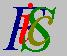Mauro Murzi's pages on Philosophy of Science - Quantum mechanics
Tunnel effectA brief recapitulation Trasmission coefficient# 4. Applications.

Two applications of Schrödinger equations are presented: the forecast of the tunnel effect and an explanation of alpha radioactivity.

## Tunnel effect.

Consider a particle, with a definite energy E, moving towards a potential barrier of height U > E: According to classical mechanics, the particle cannot go through that barrier. On the contrary, in quantum mechanics there is a not null probability that the particle goes through the barrier. This is a consequence of first Schrödinger equation.

In the following, we will study the tunnel effect with respect to a particle moving in only one direction, to simplify the problem from a mathematical point of view.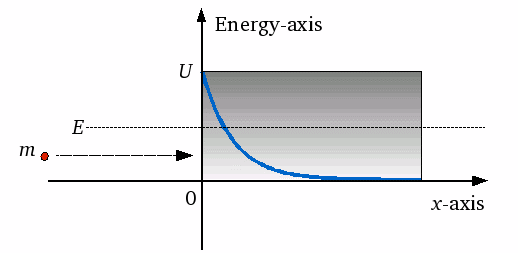A particle moving along the x-axis, with mass m and energy E, approaches from the left a potential barrier with energy U > E. According to classical mechanics, the particle cannot go inside the potential barrier: The grey area in the draw is inaccessible. According to quantum mechanics, the probability that the particle penetrates the potential barrier (blue line in the draw) is not null.

The first Schrödinger equation applied to that particle is: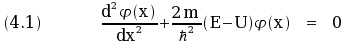Equation (4.1) is a linear differential equation with constant coefficients. The auxiliary equation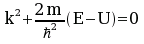has two real solutions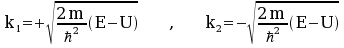.

Thus two solutions of equation (4.1) are the real functions φ1(x) = ex k and φ2(x) = e- x k, where e is is the base of natural logarithms. Since the first divergent solution φ1(x) is not acceptable, the general solution for equation (4.1) is the real function φ(x) = A · e- x  k, where A is a real arbitrary constant and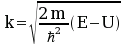.

The function φ(x) = A · e - x k describe the state of the particle inside the potential barrier. The square of its module gives the probability P(x) that the particle is in a point x occupied by the potential barrier: P(x) = | A · e- x k | 2 = A2 · e - 2 x k . The probability that the particle is in a point inside the potential barrier is not null. Thus, according to Schrödinger quantum mechanics, a particle can penetrate in a potential barrier in a manner prohibited by classical mechanics.A brief recapitulation Trasmission coefficient